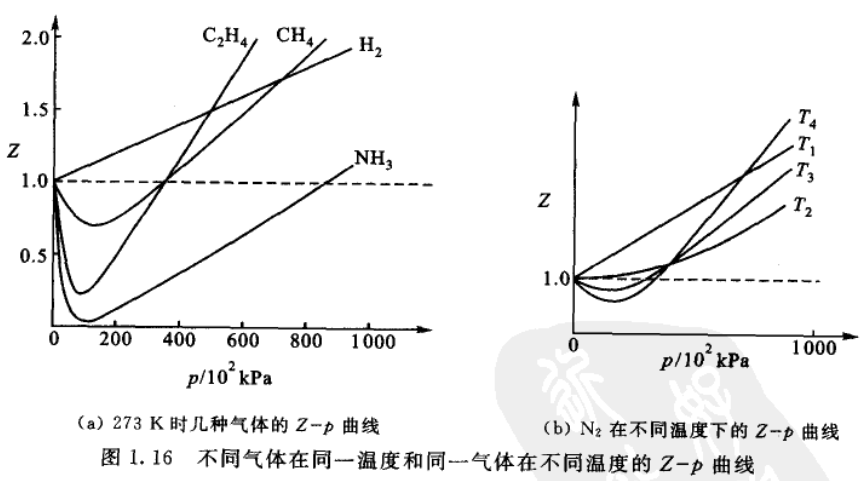## 物理化学

### 1.气体

1. 什么是等离子态（plasma state）? 答：等离子态不同于固、液、气态，等离子态是由大量带正负电荷的粒子、电子以及少量未被电离的分子、原子等，是一种新的导电流体，但是整体还是呈现电中性。如闪电、极光等。

2. 理想气体状态方程和Dalton气体分压定律？

3. 摩尔气体常数R的测量？

4. 理想气体状态图等温线（isotherm）；等压线（isobar）

5. Maxwell速率分布定律是什么？6. 分子运动速率的几个重要统计平均值？

7. 什么是分子射线束实验？金属气体蒸汽，从A中小孔射出，然后经过定向狭缝S，改变S1和S2的转速，就可以测量不同速率的蒸汽。
概率论中指出，测定某一速率范围的分子数的统计平均值为$$\Delta n$$，那么实际分子数对于这个统计平均值的偏移范围即涨落幅度基本上是$$\pm \sqrt{\Delta n}$$，所以分子数越多，统计的相对误差就越小，得到的速率分布就更接理论值。

8. 分子平均动能分布式怎样的？通常在物理化学中，都假定分子在二维平面运动，这样简化之后，可以得到
$$\frac{N_{E_{1} \rightarrow \infty}}{N}=e^{-\frac{E_{1}}{k T}}$$也就是能量超过E1的分子的比例，继续延伸，可以得如下关系式：$$\frac{N_{E_{2} \rightarrow \infty}}{N_{E_{1} \rightarrow \infty}}=e^{-\frac{\left(E_{2}-E_{l}\right)}{k T}}=e^{-\frac{\Delta E}{R T}}$$这和玻尔兹曼分布很像（在两个能量状态都的简并度g相等的情况）

9. 重力场对气体分子分布的影响？

10. 什么是气体分子碰撞的平均自由程？不同分子的碰撞与化学反应的速率直接有关。

11. 实际气体与理想气体状态方程的关系？图(b)中$$T_4$$的温度最低，$$T_1$$的温度最高。温度为$$T_2$$时，此时曲线以较缓的趋势趋向于水平线，并与水平线$$Z=1$$相切。此时在相当一段压力范围内$$Z \approx 1$$，随压力变化不大，并符合理想气体状态方程。此时的温度称为波义尔温度，图形上表现为在此温度时，等温线的坡度等于零，即（1）压力较大的时候，实际气体分子的体积无法忽略，所以引入纠正系数b，也就是实际体积是原来的体积减去气体分子所占的体积$$b$$（见图1.13(b)）。
（2）对于靠近容器壁的分子来说，后面的分子对它施加吸引力，趋向于把分子向后拉，所以气体施于器壁的压力要比忽略引力时（看作理想气体）小。这一部分的大小和容器内的气体分子数目成正比，又和碰撞的分子数目成正比，两个数目都是N，其实最终就是和密度的平方成反比。上式是气体为1mol时候的方程。实际是如下：
$$\left(p+\frac{a n^{2}}{V^{2}}\right)(V-n b)=n R T$$

12. 气体和液体之间的转变？
13. 压缩因子图？

### 2.热力学

1. 基本内容简介？

2. 冬天，在户外，手去触摸铁器和触摸木材，感受到的温度不一样，为什么？

3.  什么是热力学第零定律？第零定律→“温度”的概念，指出了温度这个状态函数的存在，而且可以用导热实验来判断不同物体的温度大小。

4. 热力学的一些基本概念？5. 什么是卡路里？

6. 什么是热力学第一定律？

1. 物体内能的增加等于物体吸收的热量和对物体所作的功的总和。
2. 系统在绝热状态时，功只取决于系统初始状态和结束状态的能量，和过程无关。
3. 孤立系统的能量永远守恒。
4. 系统经过绝热循环，其所做的功为零，因此第一类永动机是不可能的（即不消耗能量做功的机械）。

$$\Delta U=U_{z}-U_{1}=Q+W\\ \mathrm{d} U=\delta Q+\delta W$$

7. 准静态过程？假定上述是一个恒温过程$$\delta W=-F_{e} \mathrm{d} l=-\left(\frac{F_{\mathrm{e}}}{A}\right)(A \mathrm{d} l)=-p_{\mathrm{e}} \mathrm{d} V$$(1) 自由膨胀（free expansion），不对外做功
(2) 外压始终维持恒定
(3) 多次（无限次）等外压膨胀上面是系统对外膨胀，下面是外界对系统做功（压缩）；右侧两个都是准静态过程，也就是说外界和系统的压力差是无限小，整个过程需要的时间是无限大。准静态过程是一个理想过程，实际上是办不到的。对于准静态过程，在膨胀过程中系统做功最大，在压缩过程环境对系统做功最小，总而言之就是使得系统能量尽可能小

8. 什么是可逆过程？

准静态过程 + 无耗散效应 = 可逆过程

9. 什么是焓(enthalpy)？

$$\Delta U=Q_V$$。

$$Q_{p}=\left(U_{2}+p V_{2}\right)-\left(U_{1}+p V_{1}\right)$$。于是定义焓：$$H \stackrel{\text { def }}{=} U+p V$$
焓并没有确切的物理意义，之所以要定义这个新的函数，纯粹是因为很实用，处理热力学化学的计算更方便。
对于等容过程，吸收的热量全部转化为内能；对于等压过程，吸收的热量全部转化为焓

10. 什么是热容？11. 内能和温度的关系？上述实验证实，对于理想气体，自由膨胀不对外做功，温度又不变，所以内能没变化。对于固定数量的气体分子来说，U由P、V、T中的两个独立变量确定，分别以T和V、T和p写成全微分的形式，比如$$\mathrm{d} U=\left(\frac{\partial U}{\partial T}\right)_{V} \mathrm{d} T+\left(\frac{\partial U}{\partial V}\right)_{T} \mathrm{d} V$$，总之，最后可以得到温度不变的时候，U对p或者V的偏导数都是0，所以得到的结论是，内能只是温度的函数，与体积和压力无关
对于实际气体，由于存在分子间作用力，所以内能与温度和体积有关（PVT三个中，只有两个独立变量）。

12.  理想气体，焓和温度的关系?

13. 等压热容和等体积热容的关系？

$$C_{p}-C_{V}=n R$$